Imagination is more important than knowledge... Albert Einstein

Guess is more important than calculation --- Knowhowacademy.com

Length - Pythagorean Theorem

For ACT
Number of Total Problems: 28.
FOR PRINT ::: (Book)

 Problem Num : 21 Type: None Section:Length Theme:None Adjustment# : 33 Difficulty: 1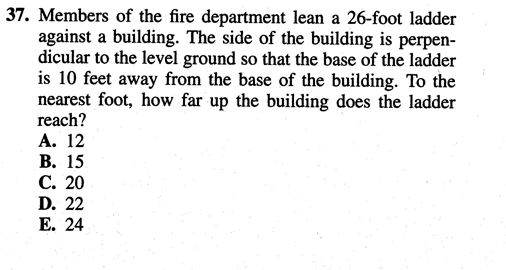Category Pythagorean Theorem
Analysis

 Problem Num : 22 Type: None Section:Length Theme:None Adjustment# : 35 Difficulty: 1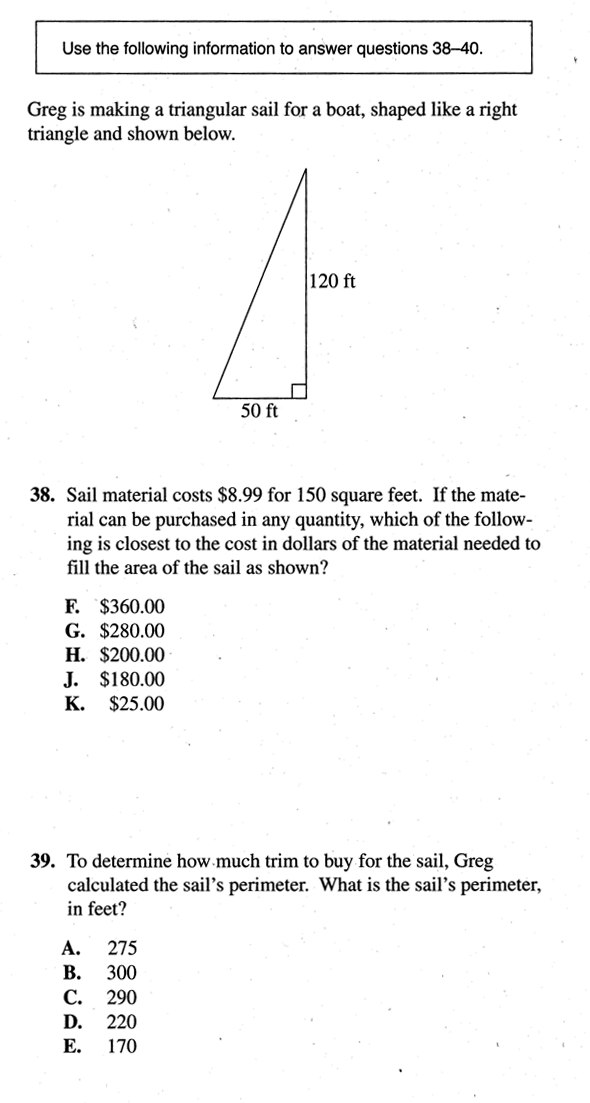Category Pythagorean Theorem
Analysis

 Problem Num : 23 Type: None Section:Length Theme:None Adjustment# : 40 Difficulty: 1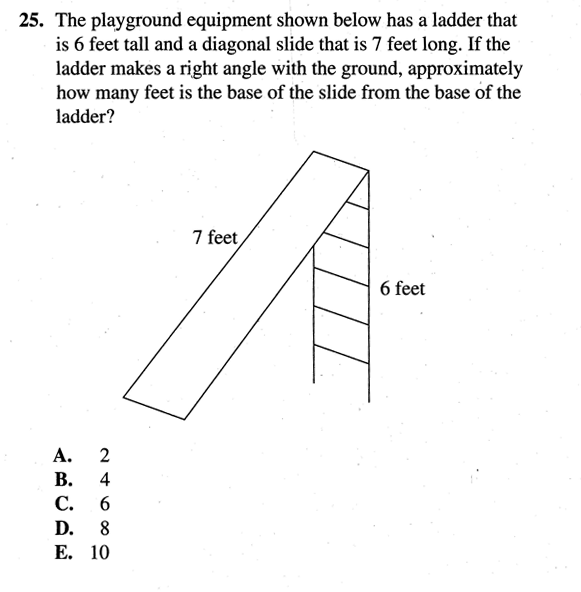Category Pythagorean Theorem
Analysis

 Problem Num : 24 Type: None Section:Length Theme:None Adjustment# : 42 Difficulty: 1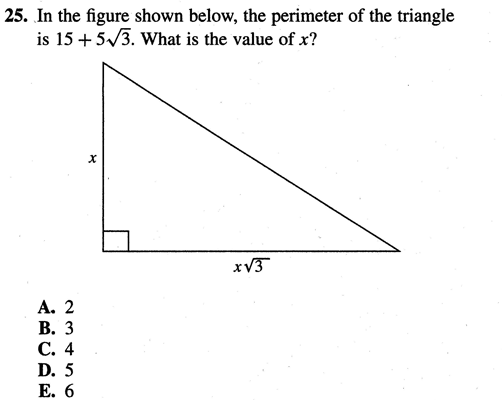Category Pythagorean Theorem
Analysis

 Problem Num : 25 Type: None Section:Length Theme:None Adjustment# : 43 Difficulty: 3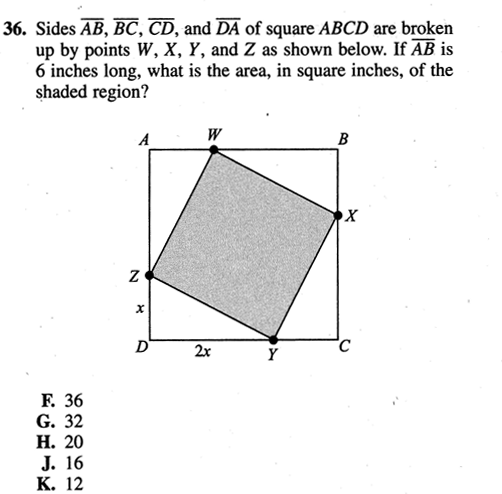Category Pythagorean Theorem
Analysis Can you guess the answer without calculation?

 Problem Num : 26 Type: None Section:Length Theme:None Adjustment# : 45 Difficulty: 1Category Pythagorean Theorem
Analysis

 Problem Num : 27 Type: None Section:Length Theme:None Adjustment# : 46 Difficulty: 1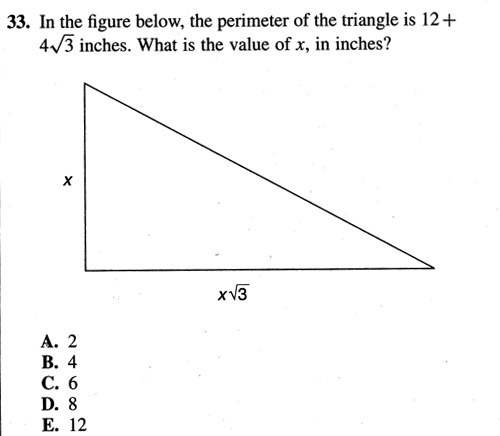Category Pythagorean Theorem
Analysis

 Problem Num : 28 Type: None Section:Length Theme:None Adjustment# : 49 Difficulty: 1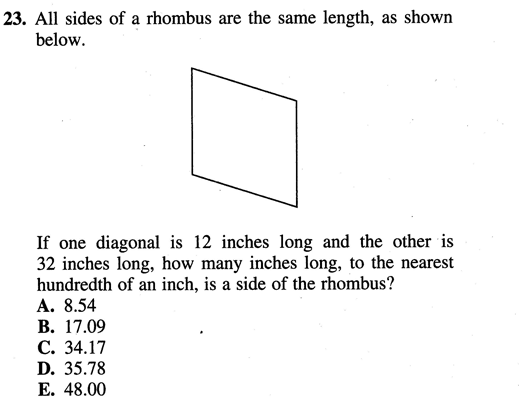Category Pythagorean Theorem
Analysis Is this a distance problem? Can you find a right triangle? Do you know the property of rhombus? What is the best way of finding a answer? Once you understand the question clearly, then u don't need any calculation sometimes!..